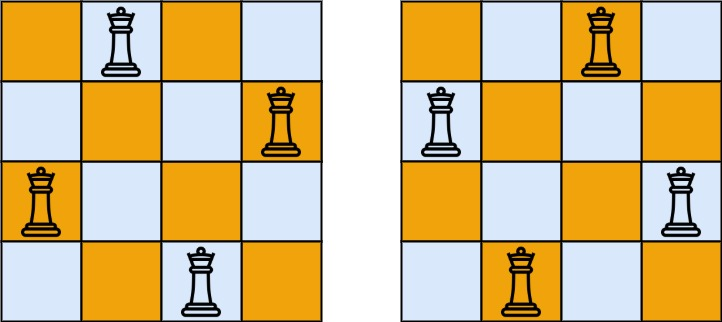## 52. N-Queens II

The n-queens puzzle is the problem of placing `n` queens on an `n x n` chessboard such that no two queens attack each other.

Given an integer `n`, return the number of distinct solutions to the n-queens puzzle.

Example 1:```Input: n = 4
Output: 2
Explanation: There are two distinct solutions to the 4-queens puzzle as shown.
```

Example 2:

```Input: n = 1
Output: 1
```

Constraints:

• `1 <= n <= 9`

## Rust Solution

``````struct Solution;

impl Solution {
fn total_n_queens(n: i32) -> i32 {
let n = n as usize;
let mut column: u32 = 0;
let mut diagonal1: u32 = 0;
let mut diagonal2: u32 = 0;
let mut res = 0;
Self::dfs(0, &mut column, &mut diagonal1, &mut diagonal2, &mut res, n);
res as i32
}
fn dfs(
i: usize,
column: &mut u32,
diagonal1: &mut u32,
diagonal2: &mut u32,
count: &mut usize,
n: usize,
) {
if i == n {
*count += 1;
} else {
for j in 0..n {
let column_bit = 1 << j;
let diagonal1_bit = 1 << (i + j);
let diagonal2_bit = 1 << (n + i - j);
if column_bit & *column == 0
&& diagonal1_bit & *diagonal1 == 0
&& diagonal2_bit & *diagonal2 == 0
{
*column |= column_bit;
*diagonal1 |= diagonal1_bit;
*diagonal2 |= diagonal2_bit;
Self::dfs(i + 1, column, diagonal1, diagonal2, count, n);
*column &= !column_bit;
*diagonal1 &= !diagonal1_bit;
*diagonal2 &= !diagonal2_bit;
}
}
}
}
}

#[test]
fn test() {
let n = 4;
let res = 2;
assert_eq!(Solution::total_n_queens(n), res);
}
``````

Having problems with this solution? Click here to submit an issue on github.